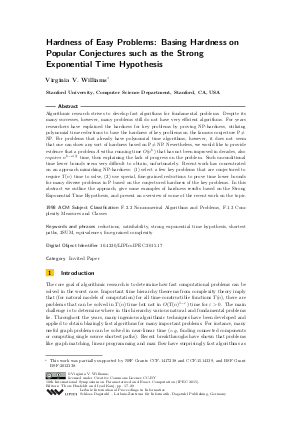Document# Hardness of Easy Problems: Basing Hardness on Popular Conjectures such as the Strong Exponential Time Hypothesis (Invited Talk)

### Author Virginia Vassilevska Williams## File

LIPIcs.IPEC.2015.17.pdf
• Filesize: 488 kB
• 13 pages

## Cite As

Virginia Vassilevska Williams. Hardness of Easy Problems: Basing Hardness on Popular Conjectures such as the Strong Exponential Time Hypothesis (Invited Talk). In 10th International Symposium on Parameterized and Exact Computation (IPEC 2015). Leibniz International Proceedings in Informatics (LIPIcs), Volume 43, pp. 17-29, Schloss Dagstuhl - Leibniz-Zentrum für Informatik (2015)
https://doi.org/10.4230/LIPIcs.IPEC.2015.17

## Abstract

Algorithmic research strives to develop fast algorithms for fundamental problems. Despite its many successes, however, many problems still do not have very efficient algorithms. For years researchers have explained the hardness for key problems by proving NP-hardness, utilizing polynomial time reductions to base the hardness of key problems on the famous conjecture P != NP. For problems that already have polynomial time algorithms, however, it does not seem that one can show any sort of hardness based on P != NP. Nevertheless, we would like to provide evidence that a problem \$A\$ with a running time O(n^k) that has not been improved in decades, also requires n^{k-o(1)} time, thus explaining the lack of progress on the problem. Such unconditional time lower bounds seem very difficult to obtain, unfortunately. Recent work has concentrated on an approach mimicking NP-hardness: (1) select a few key problems that are conjectured to require T(n) time to solve, (2) use special, fine-grained reductions to prove time lower bounds for many diverse problems in P based on the conjectured hardness of the key problems. In this abstract we outline the approach, give some examples of hardness results based on the Strong Exponential Time Hypothesis, and present an overview of some of the recent work on the topic.
##### Keywords
• reductions
• satisfiability
• strong exponential time hypothesis
• shortest paths
• 3SUM
• equivalences
• fine-grained complexity

## Metrics

• Access Statistics
• Total Accesses (updated on a weekly basis)
0